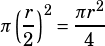What is the area of the largest circle that can be inscribed in a semicircular region of radius r?
A) (πr^2)/4
B) (πr^2)/3
C) (πr^2)/2
D) (2/3)πr^2
E) (3/4)πr^2
I don’t understand why the circle inscribed radius is half the semicircular ones?

Draw it!There’s your semicircle. What’s the biggest circle you can fit inside it? One whose diameter equals the radius of the semicircle!If the radius of the semicircle is r, then the radius of the purple circle is. Therefore, its area is